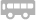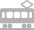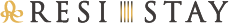##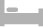房间介绍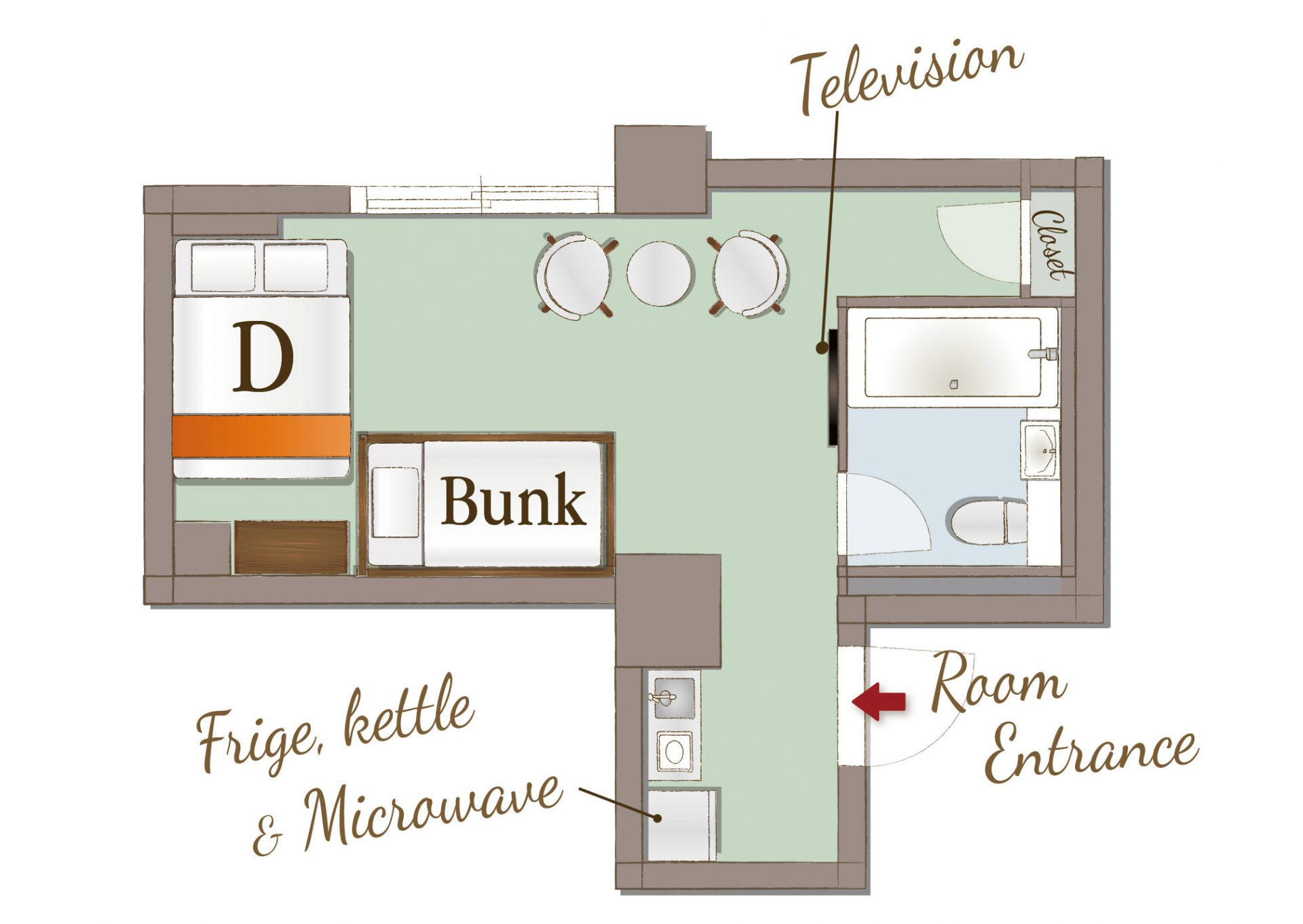## Bunk Bed with Double Bed

[可容纳人数 : 4 位]
・双层床(幅 1,100mm)×1张
・双人床(幅 1,400mm) × 1张

• 温水便座
• 浴缸
• 吹风机
• 空调
• 保险柜
• TV
• AppleTV/androidTV
• Netflix
• USB充电插口
• Wi-Fi
• 冰箱
• 微波炉
• 电水壶
• 電磁爐
• 烹调器具
• 餐具
• 公用洗衣机
• 衣架
• 电熨斗
• 壁橱
• 吸尘器
• 禁烟
• 室内禁烟(仅在阳台可吸烟)

• 洗发水
• 护发素
• 沐浴液
• 洗脸毛巾
• 浴巾
• 浴室防滑垫
• 拖鞋
• 化妆棉
• 牙刷
• 化妆棉
• 沐浴剂
• 便笺/笔
• 咖啡/茶/绿茶
• 矿泉水
• 梳子
• 洗手液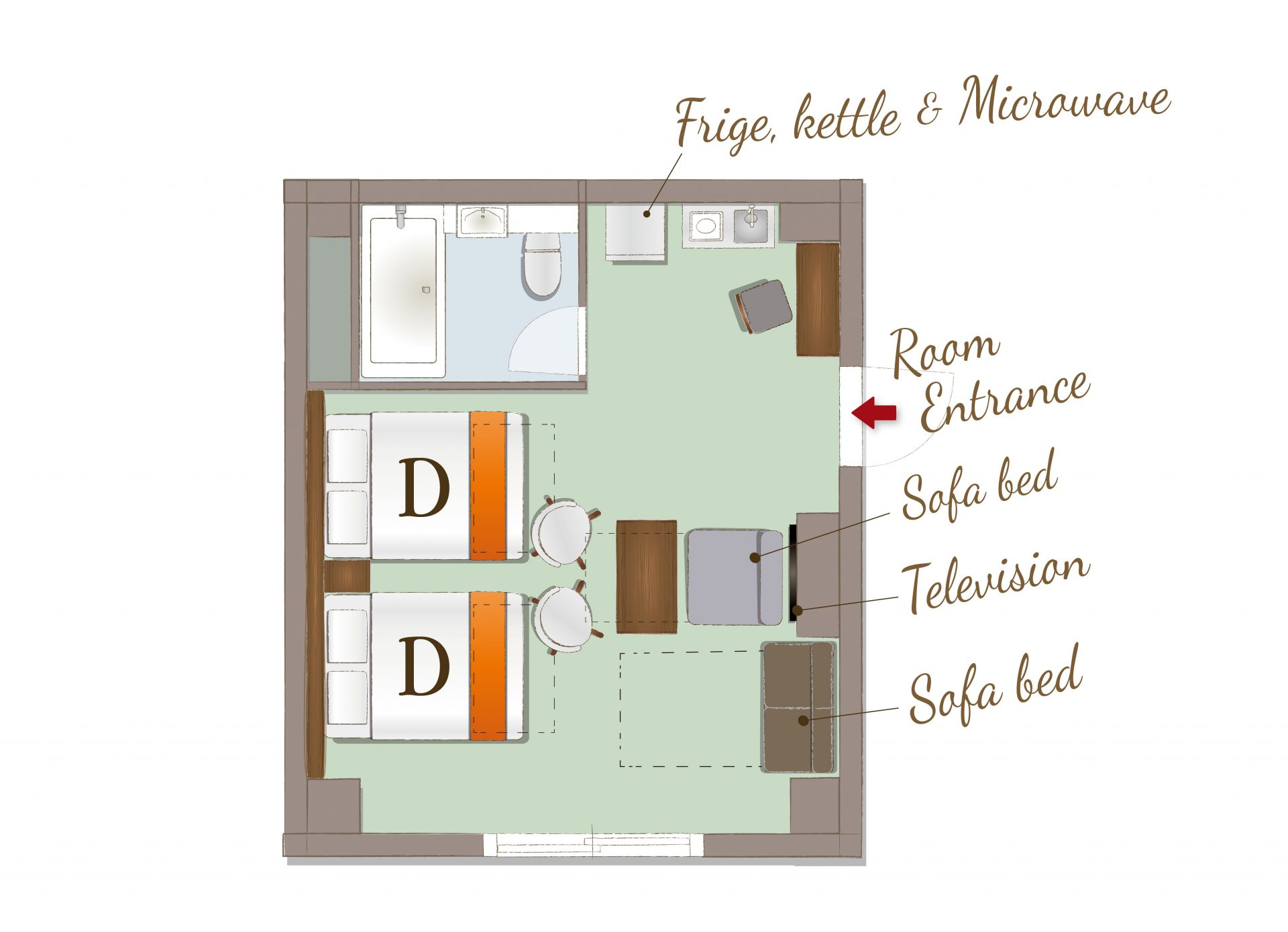## 2 Double beds with Sofa Bed

[可容纳人数 : 5 位]
・双人床(幅 1,400mm) × 2张
・沙发床(幅 1,100mm) × 1张

• 温水便座
• 浴缸
• 吹风机
• 空调
• 保险柜
• TV
• AppleTV/androidTV
• Netflix
• USB充电插口
• Wi-Fi
• 冰箱
• 微波炉
• 电水壶
• 電磁爐
• 烹调器具
• 餐具
• 公用洗衣机
• 衣架
• 电熨斗
• 壁橱
• 吸尘器
• 禁烟
• 室内禁烟(仅在阳台可吸烟)

• 洗发水
• 护发素
• 沐浴液
• 洗脸毛巾
• 浴巾
• 浴室防滑垫
• 拖鞋
• 化妆棉
• 牙刷
• 化妆棉
• 沐浴剂
• 便笺/笔
• 咖啡/茶/绿茶
• 矿泉水
• 梳子
• 洗手液## Birthday room

[可容纳人数 : 5 位]
・双人床(幅 1,400mm) × 2张
・沙发床(幅 1,100mm) × 1张

• 温水便座
• 浴缸
• 吹风机
• 空调
• 保险柜
• TV
• AppleTV/androidTV
• Netflix
• USB充电插口
• Wi-Fi
• 冰箱
• 微波炉
• 电水壶
• 電磁爐
• 厨房
• 烹调器具
• 餐具
• 公用洗衣机
• 衣架
• 电熨斗
• 壁橱
• 吸尘器
• 禁烟
• 室内禁烟(仅在阳台可吸烟)

• 洗发水
• 护发素
• 沐浴液
• 洗脸毛巾
• 浴巾
• 浴室防滑垫
• 拖鞋
• 化妆棉
• 牙刷
• 化妆棉
• 沐浴剂
• 便笺/笔
• 咖啡/茶/绿茶
• 矿泉水
• 梳子
• 洗澡巾
• 洗手液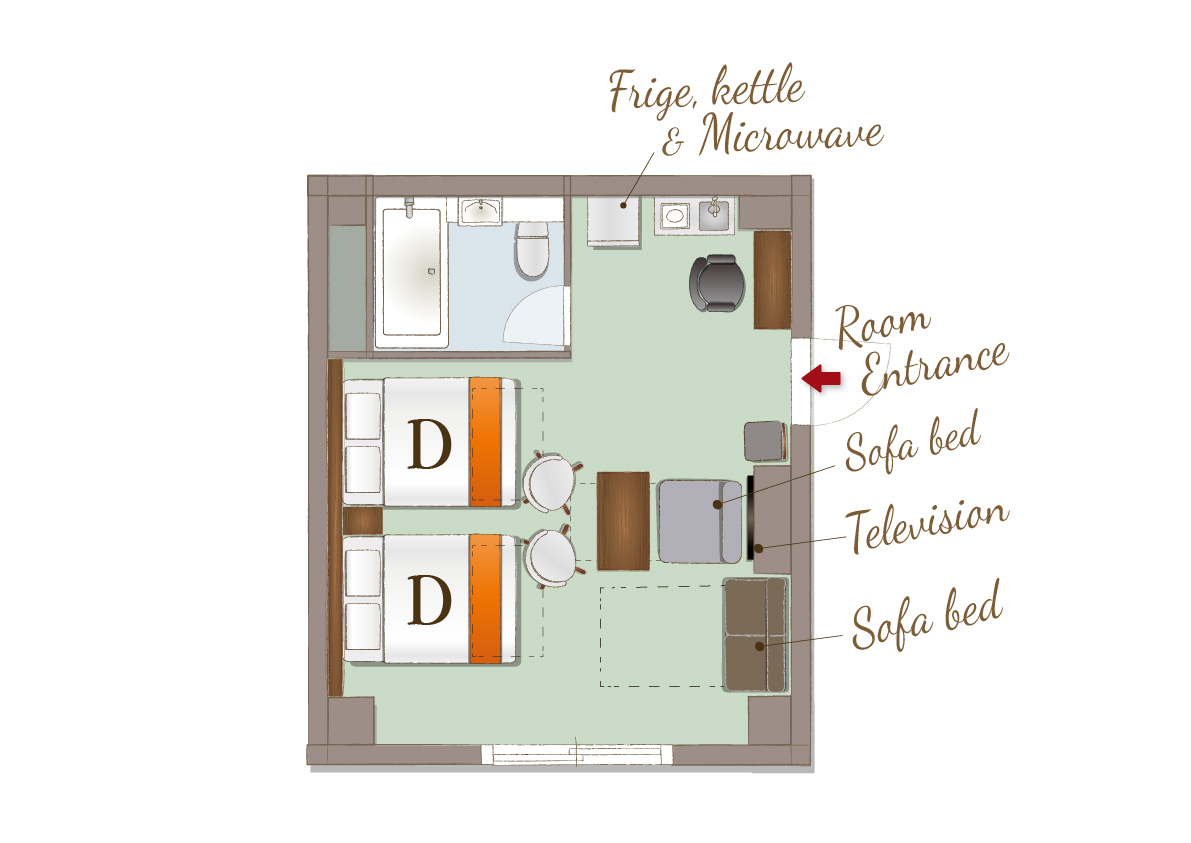## Telework room with 2 Double beds and 1 Sofa bed

[可容纳人数 : 5 位]
・双人床(幅 1,400mm) × 2张
・沙发床(幅 1,100mm) × 1张

【入住手续 15:00 / 退房手续 10:00】

• 温水便座
• 浴缸
• 吹风机
• 空调
• 保险柜
• TV
• AppleTV/androidTV
• Netflix
• USB充电插口
• Wi-Fi
• Bluetooth 扬声器
• 冰箱
• 微波炉
• 电水壶
• 電磁爐
• 烹调器具
• 餐具
• 公用洗衣机
• 衣架
• 电熨斗
• 壁橱
• 吸尘器
• 室内禁烟(仅在阳台可吸烟)
• 咖啡机

• 洗发水
• 护发素
• 沐浴液
• 洗脸毛巾
• 浴巾
• 浴室防滑垫
• 拖鞋
• 化妆棉
• 牙刷
• 化妆棉
• 沐浴剂
• 便笺/笔
• 咖啡/茶/绿茶
• 矿泉水
• 梳子
• 洗手液

##交通